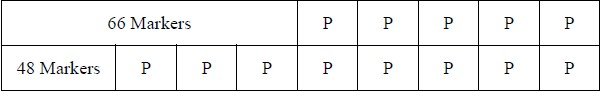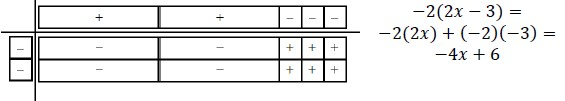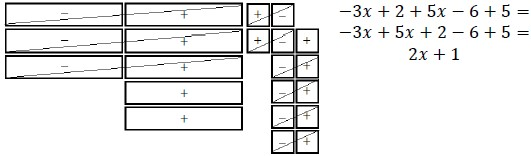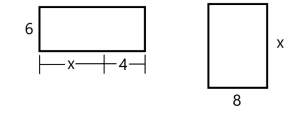# MA.912.AR.2.1Export Print
Given a real-world context, write and solve one-variable multi-step linear equations.
General Information
Subject Area: Mathematics (B.E.S.T.)
Strand: Algebraic Reasoning
Status: State Board Approved

## Benchmark Instructional Guide

### Terms from the K-12 Glossary

• Linear Equation

### Vertical Alignment

Previous Benchmarks

Next Benchmarks

### Purpose and Instructional Strategies

In grade 8, students solved one-variable multi-step linear equations. In Algebra I, students write and solve one-variable multi-step linear equations within a real-world context. In future courses, students will work with linear systems in three-variables and linear programing. Additionally, linear equations and linear functions are fundamental parts of all future high school courses.
• Problem types includes the writing of an equation from a given context, the solving of a given equation and writing and solving an equation within context.
• Instruction includes the use of manipulatives, drawings, models and the properties of equality.
• Instruction includes the interpretation of the solution within context.
• Instruction emphasizes the understanding solving a linear equation in one variable mirrors the process of determining $x$-intercepts, or roots, of the graph of a linear function.
• In many contexts, students may generate solutions that may not make sense when placed in context. Be sure students assess the reasonableness of their solutions in terms of context to check for this (MTR.6.1).
• For example, if students are solving a problem where $x$ represents the number of paintings sold at an art gallery. If the solution is $x$ = 6.3, then the number of paintings sold would be 6 since a portion of a painting cannot be sold.

### Common Misconceptions or Errors

• Students may experience difficulty translating contexts into expressions. In these cases, give students sample quantities to help them reason.
• Students may not use properties of equality properly.

### Strategies to Support Tiered Instruction

• Instruction includes opportunities to draw pictures or use bar models to represent real world contexts.
• For example, Kevin buys 66 markers plus 5 packs of markers. Fernando buys 48 markers plus 8 packs of markers. If Kevin and Fernando buy the same total number of markers, how many markers are in a pack?• Instruction includes providing expressions and having students act out the context with props.
• For example, Karen earns \$100 a day plus \$5 commission for each sale made at the store that day. In this example, give a student \$100 in play money and then ask how much more they would get if they made 1 sale, 2 sales, and so on. Then ask how they could represent an unknown amount of sales.
• Instruction includes opportunities to use algebra tiles to model a multi-step equation and write the steps algebraically. For each step, ask students to identify the property of equality they would use.
• Instruction includes vocabulary development by co-creating a graphic organizer for each property of equality.
• Instruction includes the use of algebra tiles to model the distributive property or to add and subtract like terms as a problem is solved algebraically.
• An example using algebra tiles for the expression −2(2$x$ − 3) is shown below.• An example using algebra tiles for the expression −3$x$ + 2 + 5$x$ − 6 + 5 is shown below.Instructional Task 1 (MTR.3.1, MTR.6.1, MTR.7.1
• City A has a current population of 156,289 residents and has an annual growth of 146 residents. City B has a current population of 151,293 and has an annual growth of 363 residents. To the nearest year, how many years will it take for City A and City B to have the same population?

Instructional Task 2 (MTR.3.1, MTR.6.1, MTR.7.1)

• A nutrition store starts a new membership program. Members of the program pay \$52 to join and can purchase a canister of protein powder for \$42.50. Non-members pay \$49 for a canister of protein powder. After how many canisters is the total cost the same for members as it is for non-members?

### Instructional Items

Instructional Item 1
• A group of friends decides to go out of town to a championship football game. The group pays \$185 per ticket plus a one-time convenience fee of \$15. They also each pay \$27 to ride a tour bus to the game. If the group spent \$2,771 in total, how many friends are in the group?

Instructional Item 2

• Two rectangular fields, both measured in yards, are modeled below. What value of $x$, in yards, would cause the fields to have equal areas?*The strategies, tasks and items included in the B1G-M are examples and should not be considered comprehensive.

## Related Courses

This benchmark is part of these courses.
1200310: Algebra 1 (Specifically in versions: 2014 - 2015, 2015 - 2022, 2022 and beyond (current))
1200320: Algebra 1 Honors (Specifically in versions: 2014 - 2015, 2015 - 2022, 2022 and beyond (current))
1200370: Algebra 1-A (Specifically in versions: 2014 - 2015, 2015 - 2022, 2022 and beyond (current))
1200400: Foundational Skills in Mathematics 9-12 (Specifically in versions: 2014 - 2015, 2015 - 2022, 2022 and beyond (current))
7912080: Access Algebra 1A (Specifically in versions: 2014 - 2015, 2015 - 2018, 2018 - 2019, 2019 - 2022, 2022 and beyond (current))
1200315: Algebra 1 for Credit Recovery (Specifically in versions: 2014 - 2015, 2015 - 2022, 2022 and beyond (current))
1200375: Algebra 1-A for Credit Recovery (Specifically in versions: 2014 - 2015, 2015 - 2022, 2022 and beyond (current))
7912075: Access Algebra 1 (Specifically in versions: 2014 - 2015, 2015 - 2018, 2018 - 2019, 2019 - 2022, 2022 and beyond (current))
1700600: GEAR Up 1 (Specifically in versions: 2020 - 2022, 2022 and beyond (current))
1700610: GEAR Up 2 (Specifically in versions: 2020 - 2022, 2022 and beyond (current))
1700620: GEAR Up 3 (Specifically in versions: 2020 - 2022, 2022 and beyond (current))
1700630: GEAR Up 4 (Specifically in versions: 2020 - 2022, 2022 and beyond (current))
1209315: Mathematics for ACT and SAT (Specifically in versions: 2022 and beyond (current))

## Related Access Points

Alternate version of this benchmark for students with significant cognitive disabilities.
MA.912.AR.2.AP.1: Given an equation in a real-world context, solve one-variable multi-step linear equations.

## Related Resources

Vetted resources educators can use to teach the concepts and skills in this benchmark.

## Formative Assessments

Solve for X:

Students are asked to solve a linear equation in one variable.

Type: Formative Assessment

Solve for N:

Students are asked to solve a linear equation in one variable with fractional coefficients.

Type: Formative Assessment

Solve for M:

Students are asked to solve a linear equation in one variable.

Type: Formative Assessment

Does It Follow?:

Students are asked if one linear equation follows from another that is assumed to be true.

Type: Formative Assessment

State Fair:

Students are asked to write and solve an equation that models a given problem.

Type: Formative Assessment

## Original Student Tutorial

Writing Inequalities with Money, Money, Money:

Write linear inequalities for different money situations in this interactive tutorial.

Type: Original Student Tutorial

## Perspectives Video: Teaching Idea

Programming Mathematics: Algebra, and Variables to control Open-source Hardware:

If you are having trouble understanding variables, this video might help you see the light.

Type: Perspectives Video: Teaching Idea

## Tutorial

Calculating Mixtures of Solutions:

This lecture shows how algebra is used to solve problems involving mixtures of solutions of different concentrations.

Type: Tutorial

## Video/Audio/Animation

Using Systems of Equations Versus One Equation:

When should a system of equations with multiple variables be used to solve an Algebra problem, instead of using a single equation with a single variable?

Type: Video/Audio/Animation

## MFAS Formative Assessments

Does It Follow?:

Students are asked if one linear equation follows from another that is assumed to be true.

Solve for M:

Students are asked to solve a linear equation in one variable.

Solve for N:

Students are asked to solve a linear equation in one variable with fractional coefficients.

Solve for X:

Students are asked to solve a linear equation in one variable.

State Fair:

Students are asked to write and solve an equation that models a given problem.

## Original Student Tutorials Mathematics - Grades 9-12

Writing Inequalities with Money, Money, Money:

Write linear inequalities for different money situations in this interactive tutorial.

## Student Resources

Vetted resources students can use to learn the concepts and skills in this benchmark.

## Original Student Tutorial

Writing Inequalities with Money, Money, Money:

Write linear inequalities for different money situations in this interactive tutorial.

Type: Original Student Tutorial

## Tutorial

Calculating Mixtures of Solutions:

This lecture shows how algebra is used to solve problems involving mixtures of solutions of different concentrations.

Type: Tutorial

## Video/Audio/Animation

Using Systems of Equations Versus One Equation:

When should a system of equations with multiple variables be used to solve an Algebra problem, instead of using a single equation with a single variable?

Type: Video/Audio/Animation

## Parent Resources

Vetted resources caregivers can use to help students learn the concepts and skills in this benchmark.